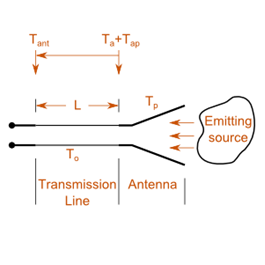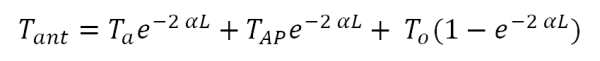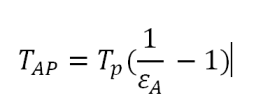#### Antenna temperature calculatorA visual representation of the antenna, transmission line and receiver connection.

Every object with a physical temperature above absolute zero radiates energy. The amount of energy radiated is usually represented by an equivalent temperature.

If an antenna maintained at a certain physical temperature (Tp) is connected to a receiver by a length of transmission line (L), with a constant temperature (To) throughout, and having uniform attenuation (α), the effective antenna temperature at the receiver terminal is:whereThe parameters used in the equation are:

Tant = antenna temperature at the receiver terminals (K)

Ta = antenna noise temperature at the antenna terminals (K)

TAP = antenna temperature at the antenna terminals due to physical temperature (K)

Tp = antenna physical temperature (K)

α = attenuation coefficient of transmission line (Np/m)

εA = thermal efficiency of the antenna

L = length of the transmission line (m)

To = physical temperature of the transmission line (K)